# Chapter 2 Visualization

Data scientists and statisticians visualize data to explore and understand data. Visualization can help analysts identify features in the data such as typical or extreme observations. Visualizations can also help describe variation. Because it is so powerful, data visualization is often the first step in any statistical analysis.

The topic of data visualization can be extremely large and entire textbooks are written about the topic.2 Given the powerful nature of data visualization, only an introduction of data visualization will be covered in this book, with the goal of using them for interpreting statistical results. As such, only a handful of data visualizations will be explored, described, and used.

Data visualization can be broken down into many different components and in many different ways. In this book, we aim to differentiate data visualization into two different types. First, in this chapter, the goal will be to explore a single attribute of interest, often referred to as univariate visualization. The term, univariate, can be split into two pieces, one, “uni-”, meaning one, and second, “variate”, referring to the attribute or variable explored. Often, univariate data visualization is used for the attribute that is deemed the outcome or the attribute of interest, but this does not need be the only attribute explored in such a way. The goals of the univariate data visualization is to understand more about the single attribute of interest, what are key features, are there extreme values, does the attribute spread over a lot of possible values or are the data more homogeneous (i.e., similar).

The second type of data visualization discussed in this book is that of multivariate data visualization. The phrase “multivariate” can again be broken into two components, “multi-” referring to more than one, and “variate”, referring to the attribute of variables. This will be discussed more in chapter 4 of this book, but multivariate visualization is used primarily to understand how more than one attribute in the data may be related to one another. Multivariate data visualization can also be useful for understanding if there are differences across smaller subgroups in the data.

This chapter will focus on univariate or single attribute data visualization. The goals for this chapter are to introduce common univariate data visualizations, how to interpret these data visualizations, and explore cases where each type of data visualization is useful to use.

### 2.0.1 College Scorecard Data

The U.S. Department of Education publishes data on institutions of higher education in their College Scorecard (https://collegescorecard.ed.gov/) to facilitate transparency and provide information for interested stakeholders (e.g., parents, students, educators). A subset of this data is provided in the file College-scorecard-clean.csv. To illustrate some of the common methods statisticians use to visualize data, we will examine admissions rates for 2,019 institutions of higher education.

Before we begin the analysis, we will load two packages, the tidyverse package and the ggformula package. These packages include many useful functions that we will use in this chapter.

library(tidyverse)
library(ggformula)

There are many functions in R to import data. We will use the function read_csv() since the data file we are importing (College-scorecard-clean.csv) is a comma separated value (CSV) file..3 CSV files are a common format for storing data. Since they are encoded as text files they generally do not take up a lot of space nor computer memory. They get their name from the fact that in the text file, each data attribute (i.e. column in the data) is separated by a comma within each row. Each row represents a unique case or observation from the data. The syntax to import the college scorecard data is as follows:

colleges <- read_csv(
file = "https://raw.githubusercontent.com/lebebr01/statthink/master/data-raw/College-scorecard-clean.csv",
guess_max = 10000
)

In this syntax we have passed two arguments to the read_csv() function. The first argument, file=, indicates the path to the data file. The data file here is stored on GitHub, so the path is specified as a URL. The second argument, guess_max=, helps ensure that the data are read in appropriately. This argument will be described in more detail later.

The syntax to the left of the read_csv() function, namely colleges <-, takes the output of the function and stores it, or in the language of R, assigns it to an object named colleges. In data analysis, it is often useful to use results in later computations, so rather than continually re-running syntax to obtain these results, we can instead store those results in an object and then compute on the object. Here for example, we would like to use the data that was read by the read_csv() function to explore it. When we want to assign computational results to an object, we use the assignment operator, <- . (Note that the assignment operator looks like a left-pointing arrow; it is taking the computational result produced on the right side and storing it in the object to the left side.)

### 2.0.2 View the Data

Once we have imported and assigned the data to an object, it is quite useful to ensure that it was read in appropriately. The head() function will give us a quick snapshot of the data by printing the first six rows of data.

head(colleges)
## # A tibble: 6 × 17
##   instnm    city   stabbr preddeg region locale adm_rate actcmmid  ugds costt4_a
##   <chr>     <chr>  <chr>  <chr>   <chr>  <chr>     <dbl>    <dbl> <dbl>    <dbl>
## 1 Alabama … Normal AL     Bachel… South… City:…    0.903       18  4824    22886
## 2 Universi… Birmi… AL     Bachel… South… City:…    0.918       25 12866    24129
## 3 Universi… Hunts… AL     Bachel… South… City:…    0.812       28  6917    22108
## 4 Alabama … Montg… AL     Bachel… South… City:…    0.979       18  4189    19413
## 5 The Univ… Tusca… AL     Bachel… South… City:…    0.533       28 32387    28836
## 6 Auburn U… Montg… AL     Bachel… South… City:…    0.825       22  4211    19892
## # … with 7 more variables: costt4_p <dbl>, tuitionfee_in <dbl>,
## #   tuitionfee_out <dbl>, debt_mdn <dbl>, grad_debt_mdn <dbl>, female <dbl>,
## #   bachelor_degree <dbl>

We can also include an interactive version for viewing the book on the web using the DT package.

DT::datatable(colleges)

## 2.1 Exploring Attributes

Data scientists and statisticians often start analyses by exploring attributes (i.e., variables) that are of interest to them. For example, suppose we are interested in exploring the admission rates of the institutions in the college scorecard data to determine how selective the different institutions are. We will begin our exploration of admission rates by examining different visualizations of the admissions rate attribute. There is not one perfect visualization for exploring the data. Each visualization has pros and cons; it may highlight some features of the attribute and mask others. It is often necessary to look at many different visualizations of the data in the exploratory phase.

One of the primary goals of any data visualization, especially those in this chapter, are to summarize (think, simplify) the data so that it can be more easily processed to understand key components of the attribute being explored. To be more explicit, it would be possible to explore all 2019 of the raw data to see the exact admission rate for each institution. However, if the goal is to know overall trends for the admission rates of institutions, knowing the exact values for each institution from the table would be too unwieldy. Instead, the goal of the data visualization to simplify the attribute to understand better the key components of the admission rate attribute. This is a trade-off, as there is a loss of information, but this loss of information is useful in this context as it allows for the summarization of the attribute.

### 2.1.1 Histograms

The first visualization we will examine is a histogram. We can create a histogram of the admission rates using the gf_histogram() function. (This function is part of the ggformula package which needs to be loaded prior to using the gf_histogram() function.) This function requires two arguments. The first argument is a formula that identifies the variables to be plotted and the second argument, data =, specifies the data object that was assigned on data import. For example, earlier we used the read_csv() function to import the college scorecard data and we assigned this to the name, colleges. The syntax used to create a histogram of the admission rates is:

gf_histogram(~ adm_rate, data = colleges)

Figure 2.1: Histogram of college admission rates

The formula we provide in the first argument is based on the following general structure:

~ attribute name

where the attribute name identified to the right of the ~ is the exact name of one of the columns in the colleges data object.

### 2.1.2 Interpretting Histograms

Histograms are created by collapsing the data into bins and then counting the number of observations that fall into each bin. To show this more clearly in the figure created previously, we can color the bin lines to highlight the different bins. To do this we include an additional argument, color =, in the gf_histogram() function. We can also set the color for the bins themselves using the fill = argument. Here we color the bin lines black and set the bin color to yellow.4

gf_histogram(~ adm_rate, data = colleges, color = 'black', fill = 'yellow')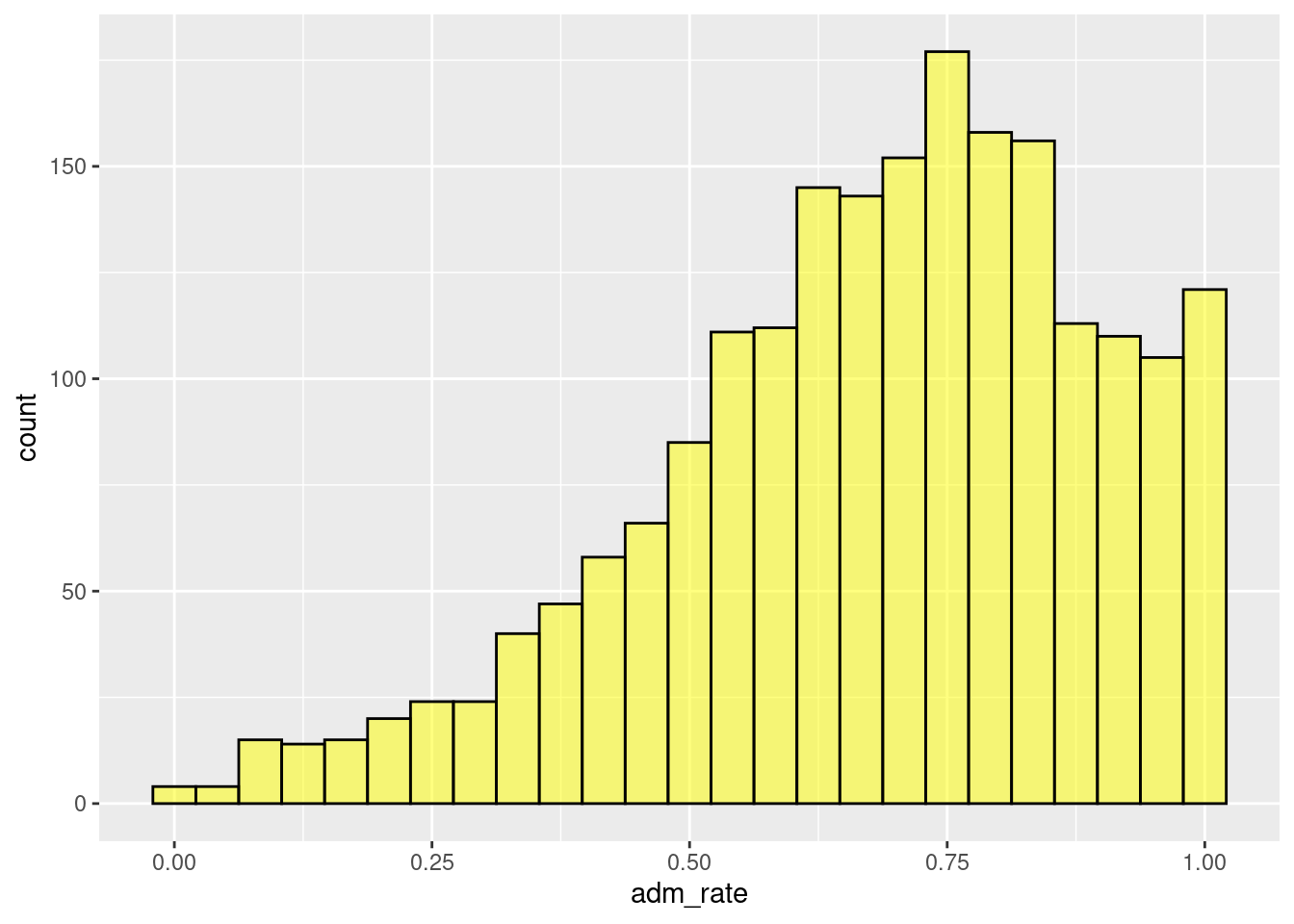Figure 2.2: Histogram changing the color and fill of the bars.

When looking at a single bar, for example the bar that is at 0.50, shows the number of institutions with admissions rates between about 0.48 and 0.52. In this case, there are about 80 institutions that have admissions rates between 0.48 and 0.52 (i.e., 48% to 52% admission rates). Similar interpretations are found for all of the other bars as well.

One common assumption made with a histogram is for the width of the bars be the same width on the attribute of interest. For example, the single bar interpreted in the preceding paragraph, the width of the bar was about 4% on the admission rate scale. Therefore, with the assumption that all bars have the same width, this would mean that all of the 30 bars in the histogram would each range by about 4%.

Rather than focusing on any one bin, we typically want to describe the distribution as a whole. For example, it appears as though most institutions admit a high proportion of applicants since the bins to the right of 0.5 have higher counts than the bins that are below 0.5. There are, however, some institutions that are quite selective, only admitting fewer than 25% of the students who apply.

#### 2.1.2.1 Adjusting Number of Bins

Interpretation of the distribution is sometimes influenced by the width or number of bins. It is often useful to change the number of bins to explore the impact this may have on your interpretation. This can be accomplished by either (1) changing the width of the bins via thebinwidth = argument in the gf_histogram() function, or (2) changing the number of bins using the bins = argument.

The binwidth in the histogram refers to the range or width of each bin. A larger binwidth would mean there are fewer bins as it would take fewer bins to span the entire range of the attribute of interest. In contrast, a smaller binwidth would require more bins to span the entire range of the attribute of interest.

In contrast, the number of bins can be specified directly, for example 10 or 20 bins. The default within the R graphics package used is 30. Within this framework, each bin will have the same width or binwidth.

The relationship between the number of bins and binwidth could be shown with the following equation:

$binwidth = \frac{attribute\ range}{\#\ of\ bins}$ To be more explicit, suppose that we wanted there to be 25 bins, using algebra we could compute the new binwidth given that we know we want 25 bins and knowing the range of the original attribute. The admission rates attribute have values as small as 0 and as large as 1. Therefore, the total range would be 1 (1 - 0 = 1). The binwidth could then be computed as:

$bindwidth = \frac{1}{25} = .04$

In contrast, if we wanted to specify the binwidth instead of the number of bins, we could do a little bit of algebra in the equation above to compute the number of bins needed to span the range of the attribute given the specified binwidth. For example, if we wanted the binwidth to be .025, 2.5%, we could compute this as follows:

$\#\ of\ bins = \frac{1}{.025} = 40$

We will take the approach of letting the software compute these, but the equations above shows the general process that is used by the software in selecting the binwidth.

More bins/smaller binwidth can give a slightly more nuanced interpretation of the attribute of interest, whereas fewer bins/large binwidth will do more summarization. Having too few bins or too many bins can make the figure more difficult to interpret by missing key features of the attribute or including too many unique features of the attribute. For this reason, it is often of interest to adjust the binwidth or number of bins to explore the impact on the interpretation.

The code below changes the binwidth to specify it as .01 via the binwidth = .01 argument with the figure shown in Figure 2.3.

gf_histogram(~ adm_rate, data = colleges, color = 'black', fill = 'yellow', binwidth = .01)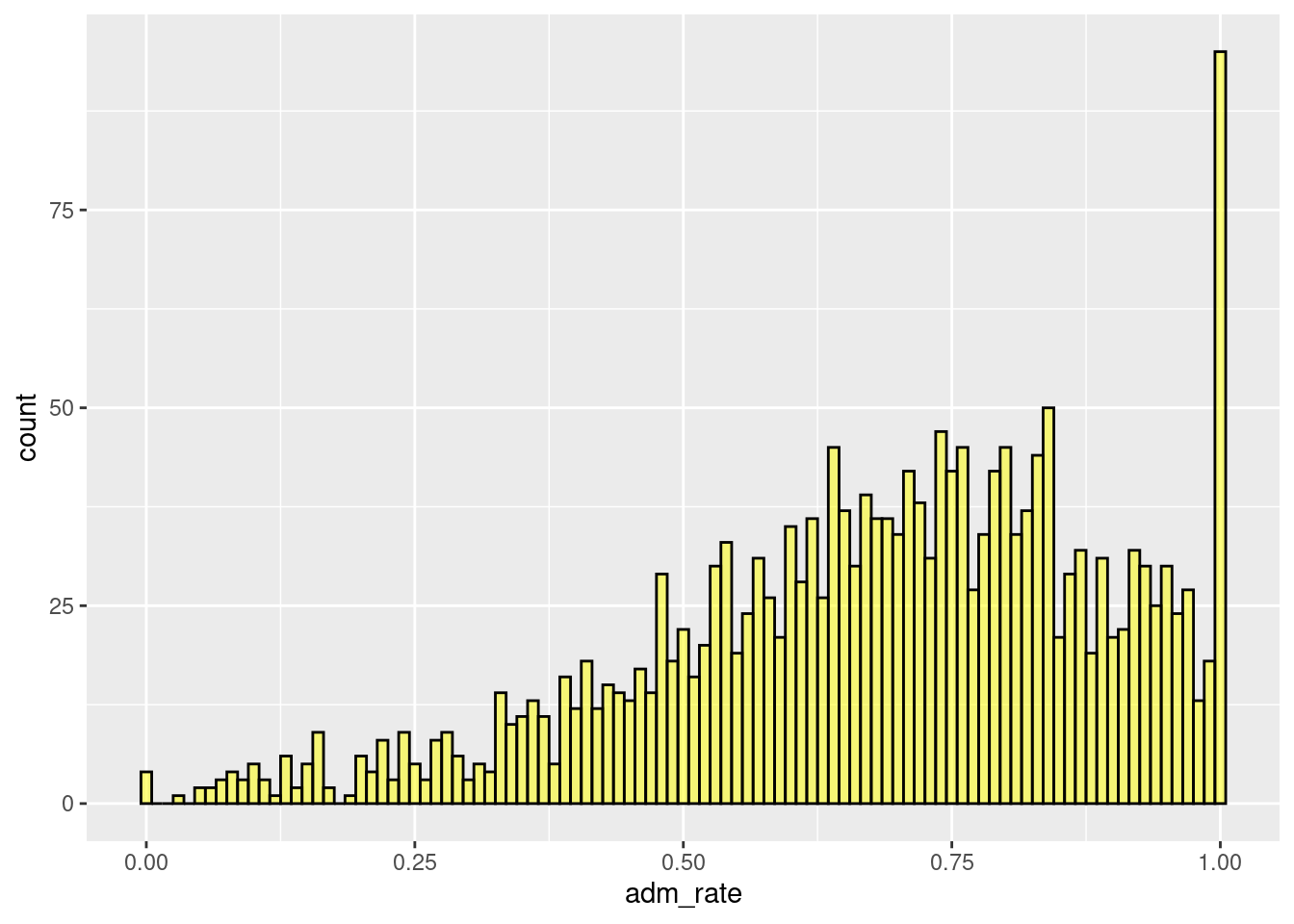Figure 2.3: Histogram modifying the binwidth.

The code below specifies 10 bins via the bins = 10 argument with the figure shown in Figure ??.

gf_histogram(~ adm_rate, data = colleges, color = 'black', fill = 'yellow', bins = 10)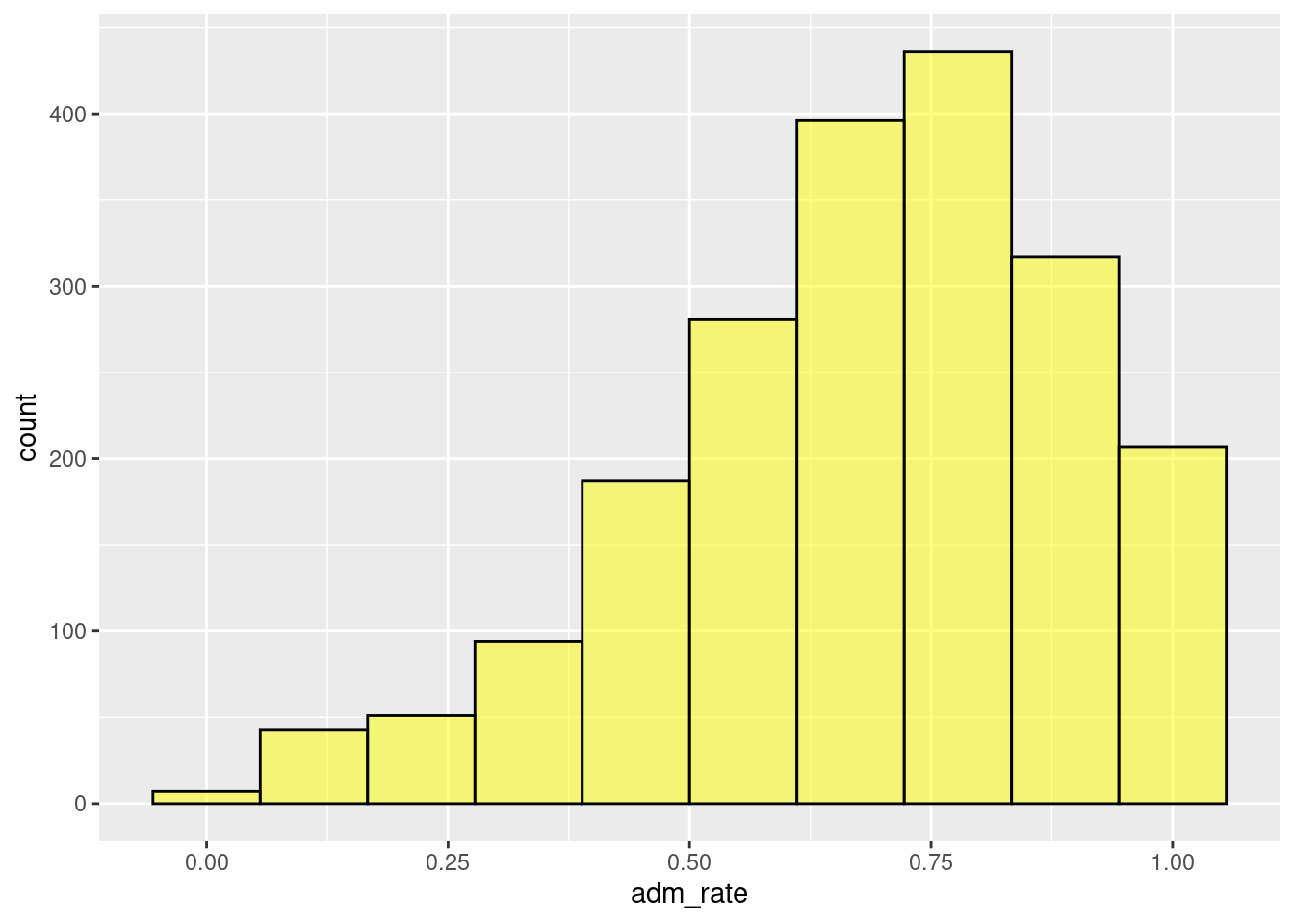Figure 2.4: Histogram modifying the number of bins.

In general, our interpretation remains the same across all the different binwidth/bins combinations, namely that most institutions admit a high proportion of applicants. When we used a bin width of 0.01, however, we were able to see that several institutions admit 100% of applicants. This was obscured in the other histograms we examined. As a data scientist these institutions might warrant a more nuanced examination.

## 2.2 Plot Customization

There are many ways to further customize the plot we produced to make it more appealing. For example, you would likely want to change the label on the x-axis from adm_rate to something more informative. Or, you may want to add a descriptive title to your plot. These customizations can be specified using the gf_labs() function. Specific examples are given below.

### 2.2.1 Axes labels

To change the labels on the x- and y-axes, we can use the arguments x = and y = in the gf_labs() function. These arguments take the text for the label you want to add to each axis, respectively. Here we change the text on the x-axis to “Admission Rate” and the text on the y-axis to “Frequency”. The gf_labs() function is connected to the histogram by linking the gf_histogram() and gf_labs() functions with the pipe operator (%>%).5

gf_histogram(~ adm_rate, data = colleges, color = 'black', fill = 'yellow', bins = 25) %>%
gf_labs(
y = 'Frequency'
)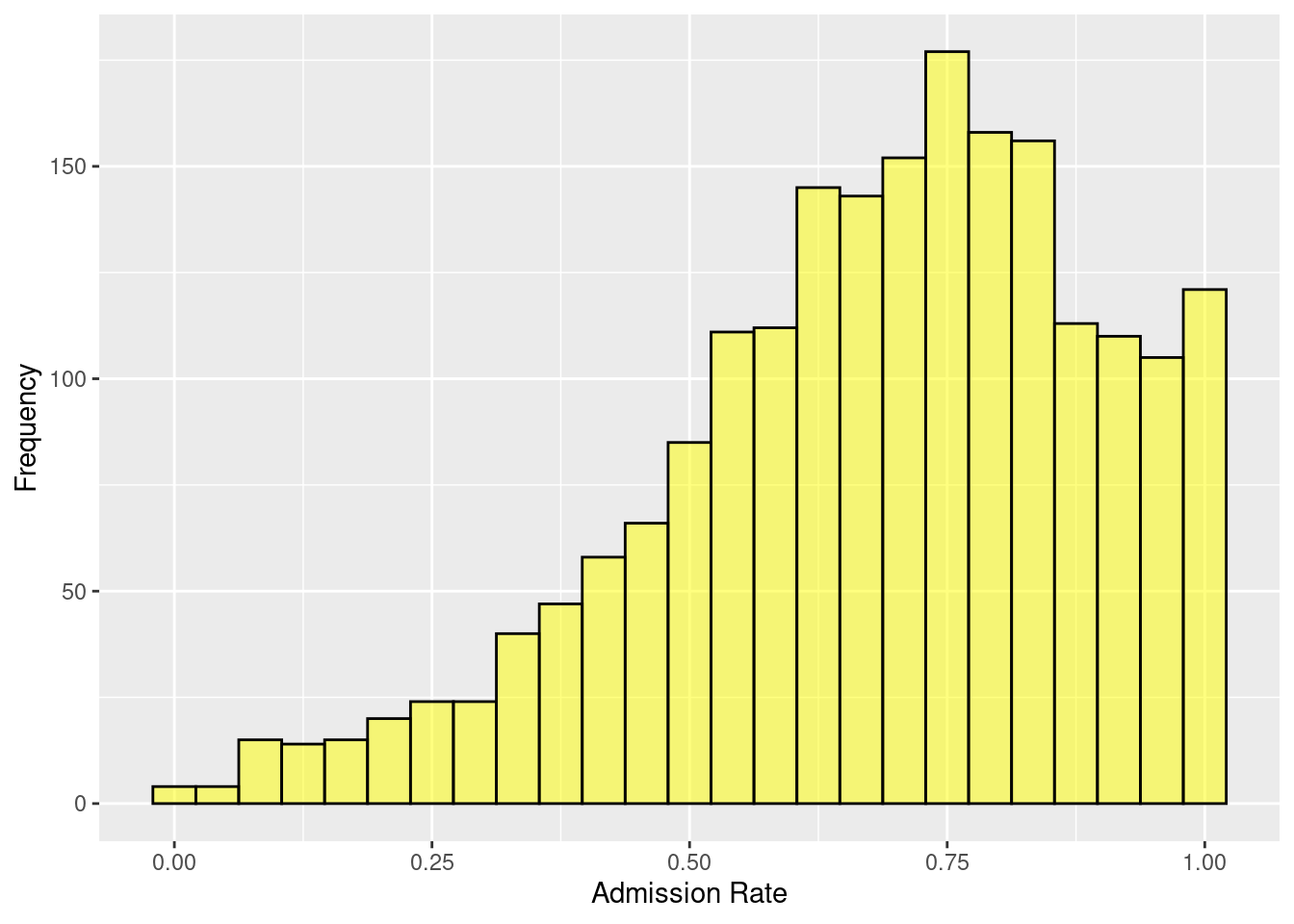Figure 2.5: Adding custom x and y-axis labels.

### 2.2.2 Plot title and subtitle

We can also add a title and subtitle to our plot. Similar to changing the axis labels, these are added using gf_labs(), but using the title = and subtitle = arguments.

gf_histogram(~ adm_rate, data = colleges, color = 'black', fill = 'yellow', bins = 25) %>%
gf_labs(
y = 'Frequency',
title = 'Distribution of admission rates for 2,019 institutions of higher education.',
subtitle = 'Data Source: U.S. Department of Education College Scorecard'
)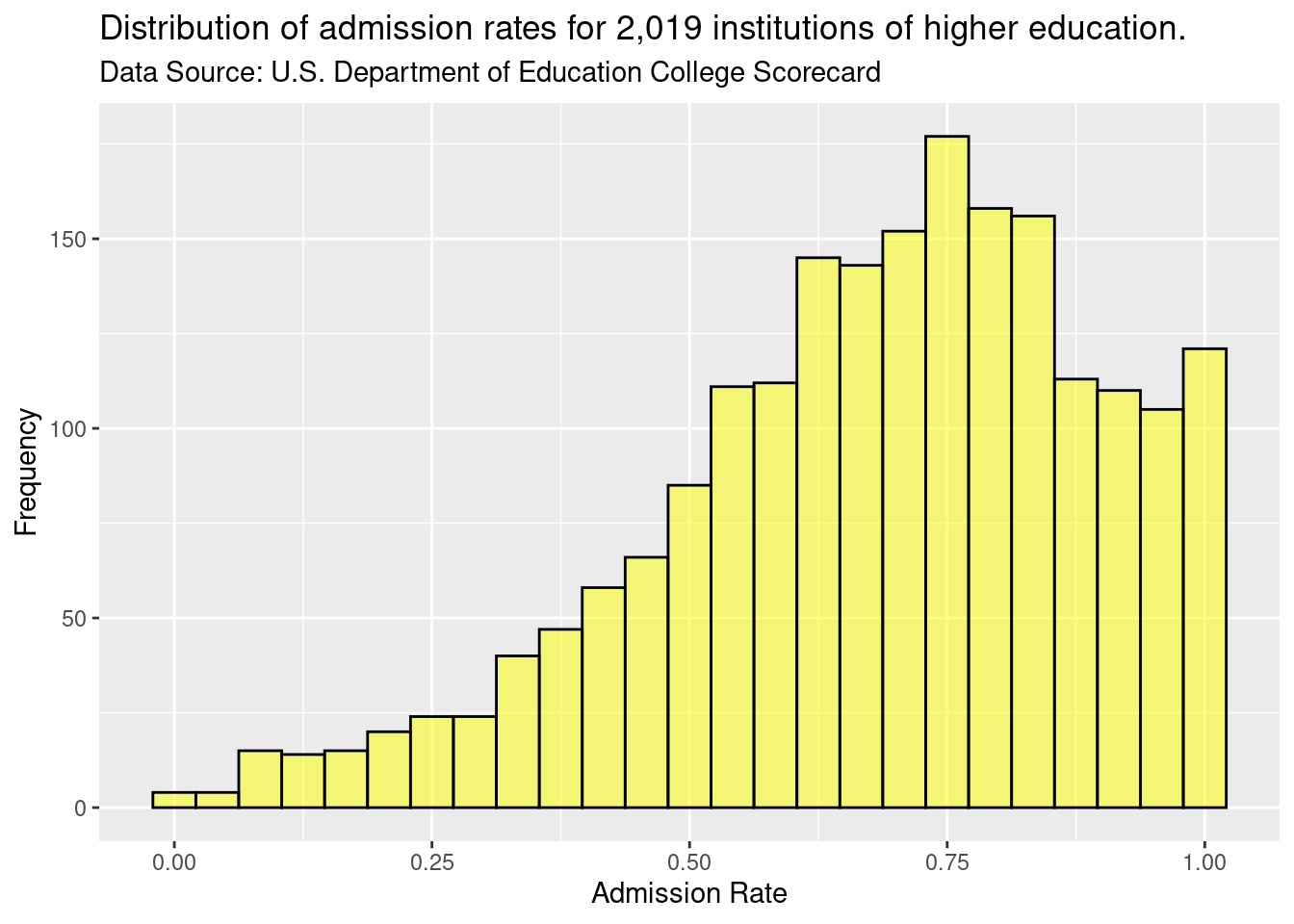Figure 2.6: Adding plot title and subtitle.

Plot titles and subtitles are helpful to used to provide context to the figure and describe the overall purpose for the figure. For example, the subtitle in Figure 2.6 describes the source for the data plotted.

### 2.2.3 Plot theme

By default, the plot has a grey background and white grid lines. This can be modified to using the gf_theme() function. For example, in the syntax below we change the plot theme to a white background with no grid lines using theme_classic(). Again, the gf_theme() is linked to the histogram with the pipe operator.

gf_histogram(~ adm_rate, data = colleges, color = 'black', fill = 'yellow', bins = 25) %>%
gf_labs(
y = 'Frequency',
title = 'Distribution of admission rates for 2,019 institutions of higher education.',
subtitle = 'Data Source: U.S. Department of Education College Scorecard'
) %>%
gf_theme(theme_classic())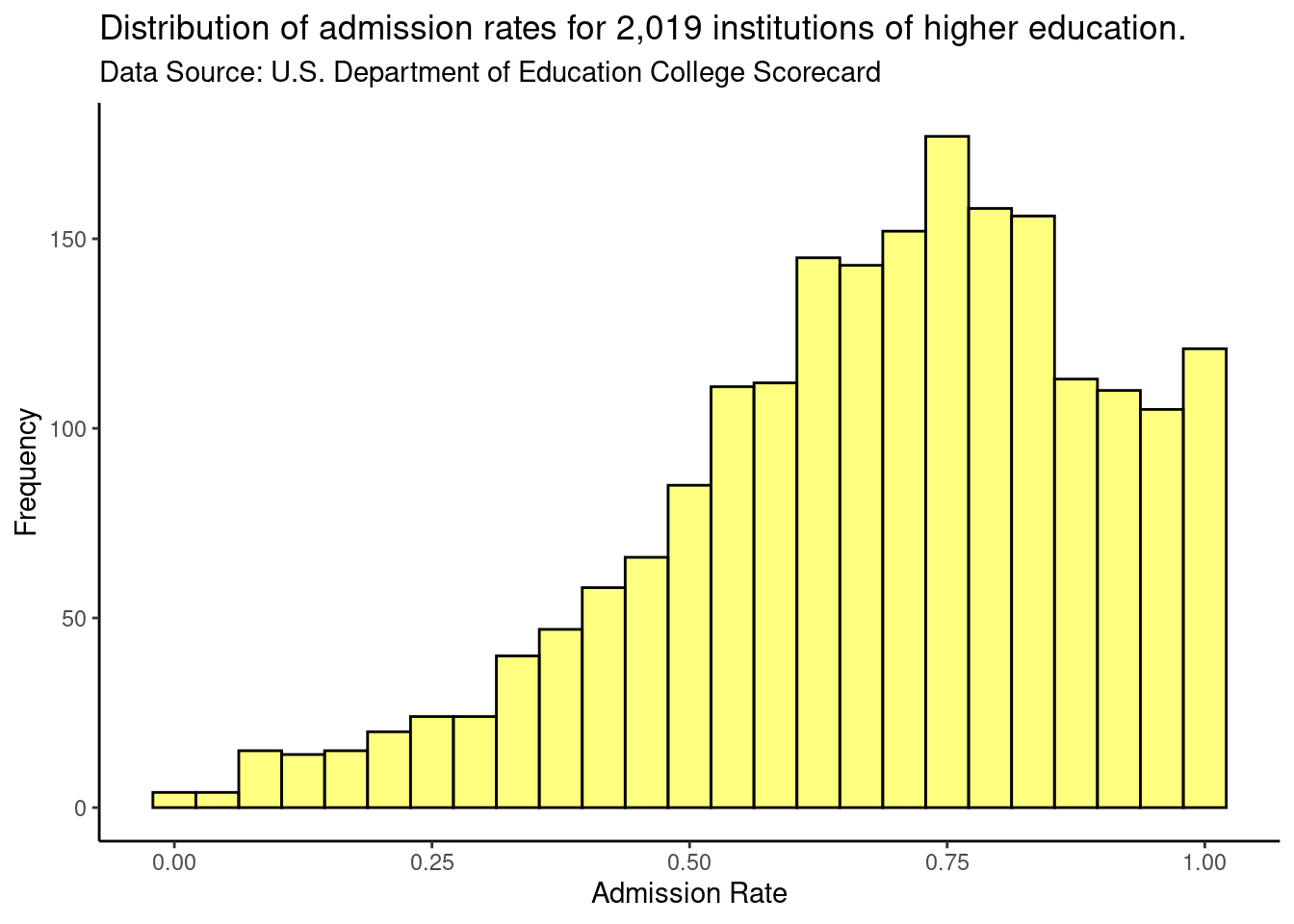Figure 2.7: Varying figure theme.

We have created a custom theme to use in the gf_theme() function that we will use for most of the plots in the book. The theme, theme_statthinking() is included in the statthink package, a supplemental package to the text that can be installed and loaded with the following commands:

remotes::install_github('lebebr01/statthink')
library(statthink)
##
## Attaching package: 'statthink'
## The following object is masked _by_ '.GlobalEnv':
##
##     colleges

We can then change the theme in a similar manner to how we changed the theme before.

gf_histogram(~ adm_rate, data = colleges, color = 'black', bins = 25) %>%
gf_labs(
y = 'Frequency',
title = 'Distribution of admission rates for 2,000 institutions of higher education.',
subtitle = 'Data Source: U.S. Department of Education College Scorecard'
) %>%
gf_theme(theme_statthinking())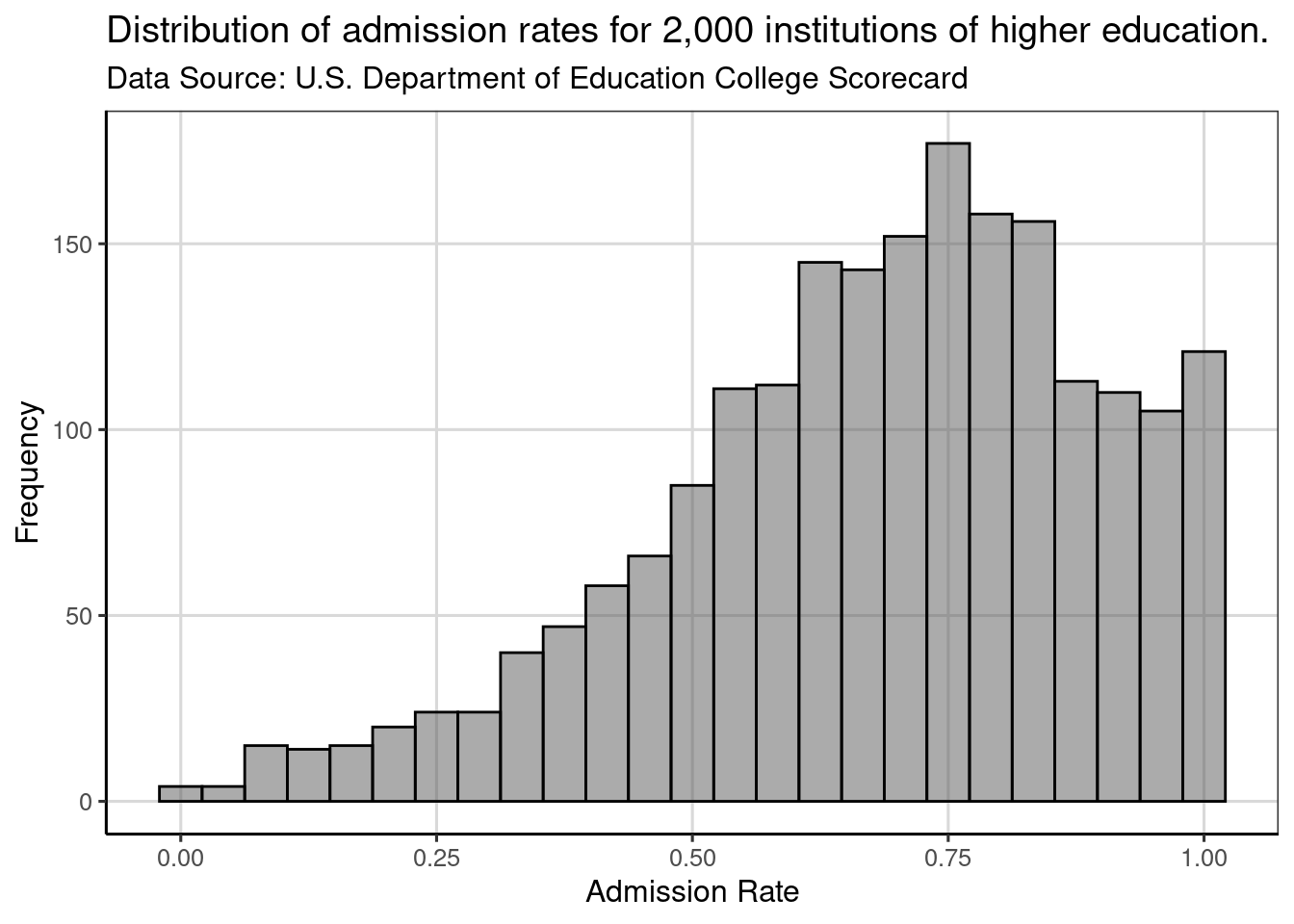Figure 2.8: Histogram showing distribution of admission rates for institutions of higher education.

#### 2.2.3.1 Setting the default plot theme

Since we will be using this theme for all of our plots, it is useful to make it the default theme (rather than the grey background with white gridlines). To set a different theme as the default, we will use the theme_set() function and specify the theme_statthinking() theme as the argument within this function.

theme_set(theme_statthinking(base_size = 14))

Now when we create a plot, it will automatically use the statthinking theme without having to specify this in the gf_theme() function. Often, the plot theme would be one of the first lines of code for any analysis so that all the figures created in subsequent areas would all have a similar theme/style.

In the code chunk above, the size of the text is increased slightly to make it a bit easier to read throughout the book. This was done with the base_size = argument, where the font size was specified to be size 14.

Figure 2.9 shows that the theme is now applied automatically without needing to use the gf_theme() function to specify. In addition, the font size is increased as well compared to previous figures created in this chapter.

gf_histogram(~ adm_rate, data = colleges, color = 'black', bins = 25) %>%
gf_labs(
y = 'Frequency',
title = 'Distribution of admission rates for 2,000 institutions of higher education.',
subtitle = 'Data Source: U.S. Department of Education College Scorecard'
)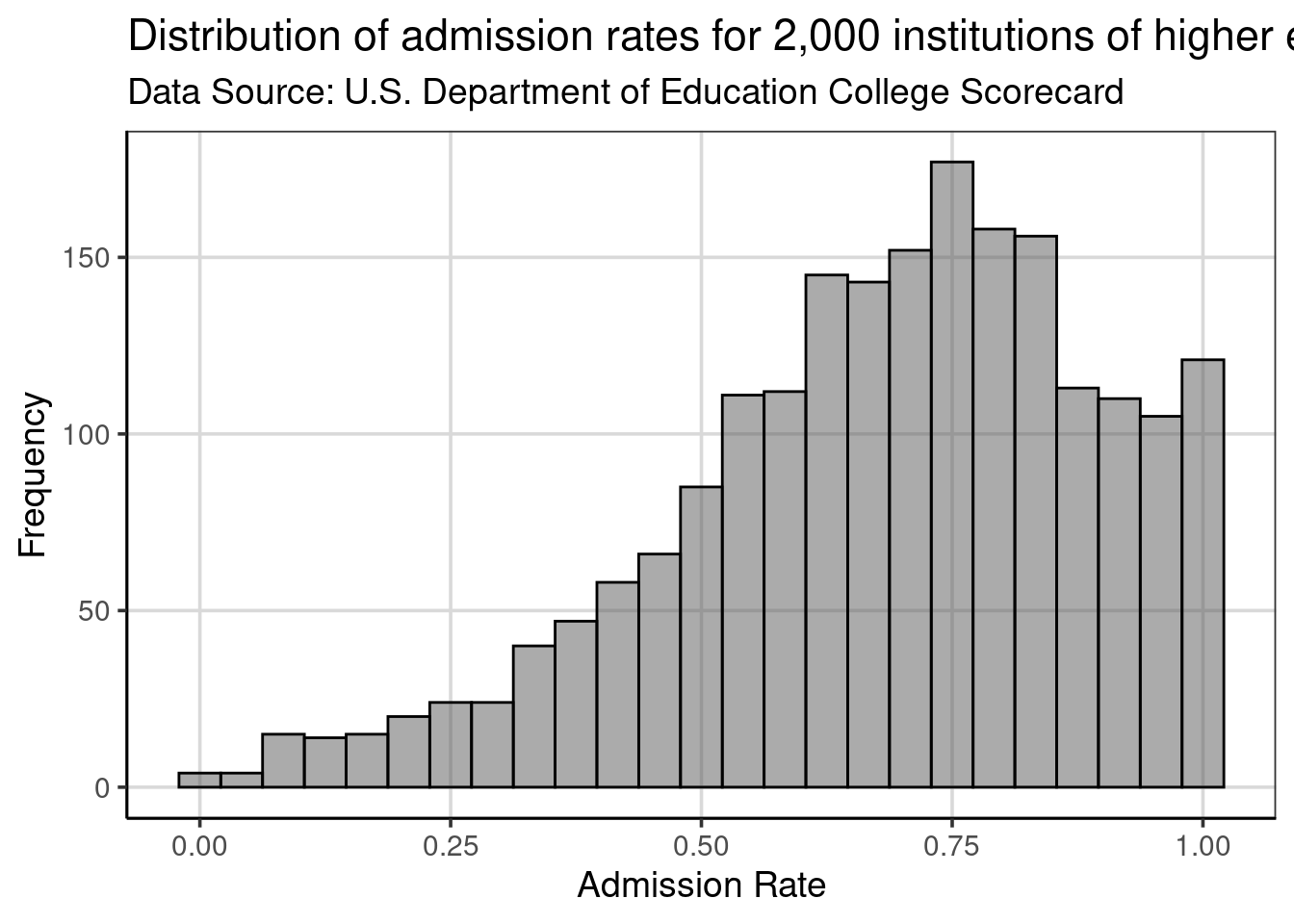Figure 2.9: Histogram showing the distribution of admission rates for institutions of higher education.

## 2.3 Density plots

Another plot that is useful for exploring attributes is the density plot. This plot usually highlights similar distributional features as the histogram, but the visualization does not have the same dependency on the specification of bins. Density plots can be created with the gf_density() function which takes similar arguments as gf_histogram(), namely a formula identifying the attribute to be plotted and the data object.6 If you compare the code specified for the very first histogram, notice that only the function name changed.

gf_density(~ adm_rate, data = colleges)Figure 2.10: Density curve of admission rates for institutions of higher education.

### 2.3.1 Interpreting Density Plots

Density plots are interpreted similarly to a histogram in that areas of the density curve that are higher indicate more data in those areas of the attribute of interest. Places where the density curve are lower indicate areas where data occur infrequently. The density metric on the y-axis is not the same as the histogram, but the relative magnitude can be interpreted similarly. That is, higher indicates more data in that region of the attribute.

Just like the histogram, the attribute being depicted in the density curve is on the x-axis. Therefore, important features for the attribute of interest can be found by looking at the y-axis, but then the place where high or low prevalence occurs are depicted by looking back to the x-axis. For example, when looking at the density curve in Figure 2.11, the density curve has a peak on the y-axis density scale of just under 2.0, the peak of this density curve occurs around a 0.75 as shown on the x-axis.

Our interpretation remains that most institutions admit a high proportion of applicants. In fact, colleges that admit around 75% of their applicants have the highest probability density, indicating this is where most of the institutions are found in the distribution. Additionally, there are just a few institutions that are have an admission rate 25% or less.

The axis labels, title, subtitle can be customized with gf_labs() in the same manner as with the histogram. The color = and fill = arguments in gf_density() will color the density curve and area under the density curve, respectively.

gf_density(~ adm_rate, data = colleges, color = 'black', fill = 'yellow') %>%
gf_labs(
y = 'Probability density',
title = 'Distribution of admission rates for 2,019 institutions of higher education.',
subtitle = 'Data Source: U.S. Department of Education College Scorecard'
)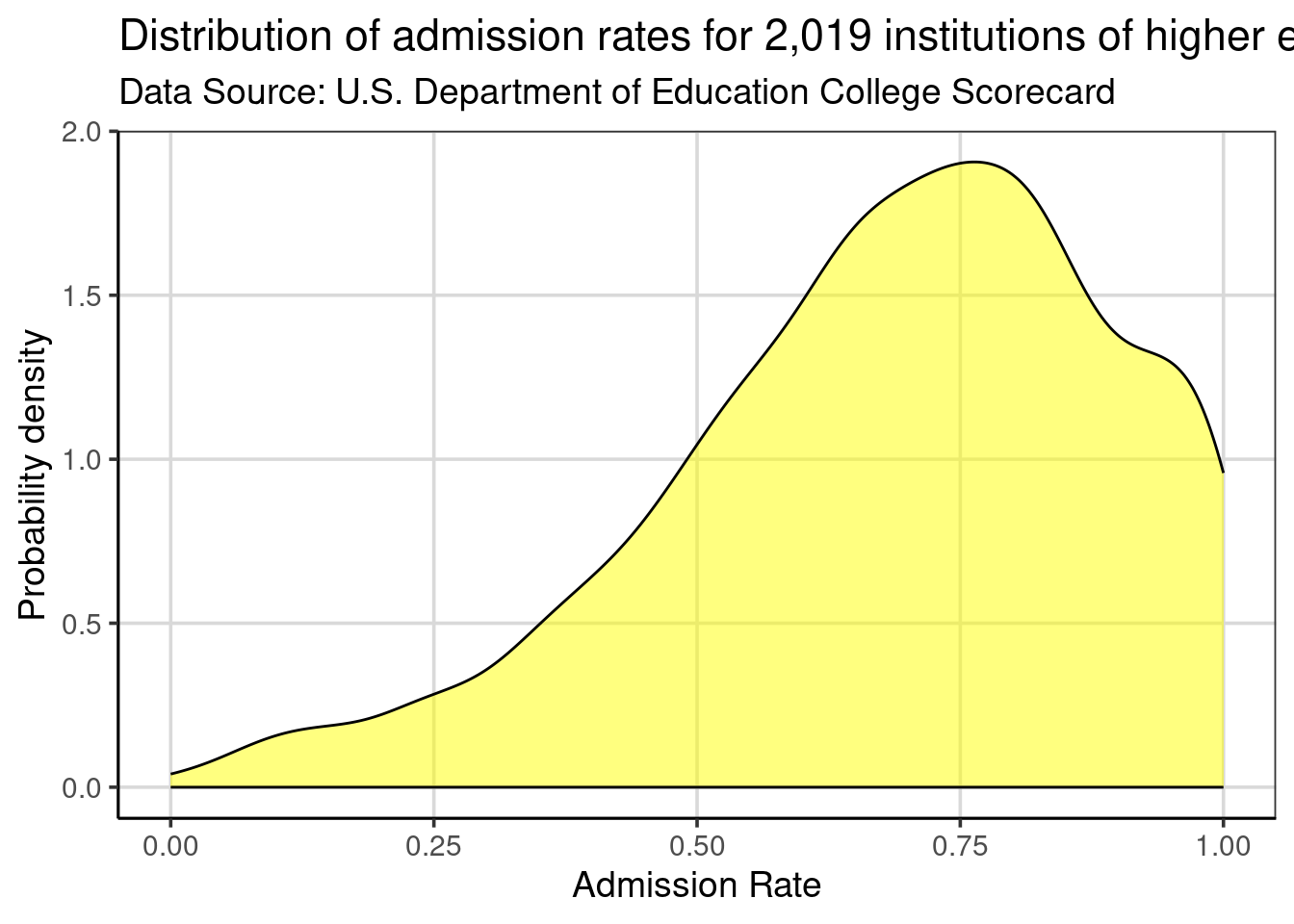Figure 2.11: Density curve showing the distribution of admission rates for institutions of higher education.

1. Clause Wilke has a great book on the Fundamentals of Data Visualization and Kieran Healy has another great book data visualization called Data Visualization: A practical introduction. Both of these books are also freely available online with print versions able to be purchased as well.↩︎

2. This function is a part of the tidyverse package, so you need to be sure to run library(tidyverse) prior to using read_csv().↩︎

3. R knows the names of 657 colors. To see these names type colors() at the command prompt.↩︎

4. The pipe operator, %>%, can be read as “then” and the code can be thought of as logical processes. For example, first the histogram is created, then (%>%), the labels for the x- and y- axes are changed.↩︎

5. The default kernel used in gf_density() is the normal kernel. This can be changed if desired, but the default works well in many situations.↩︎